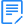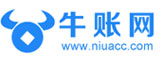# Excel反向查找、交叉查找公式是什么？

Excel反向查找、交叉查找公式是什么？

1、普通查找

=VLOOKUP(H2,B:F,5,0)2、反向查找

=INDEX(A:A,MATCH(H2,B:B,0))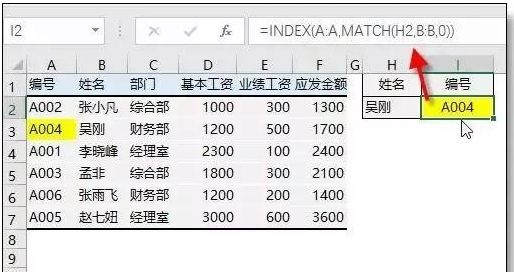3、交叉查找

=VLOOKUP(H2,A:F,MATCH(I2,1:1,0),0)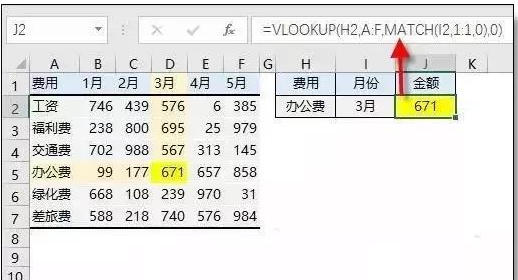4、多条件查找

=LOOKUP(1,0/((A2:A7=E2)*(B2:B7=F2)),C2:C7)5、区间查找

=LOOKUP(A2,\$D\$2:\$E\$5)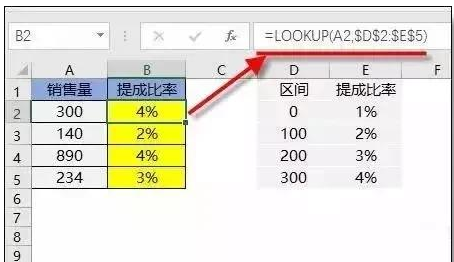## 限时资料免费

•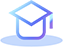重点汇总

•历年真题

•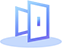章节练习

•备考攻略

•高分经验

•押题密卷

## 推荐课程